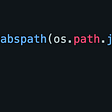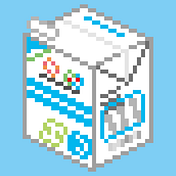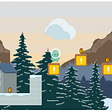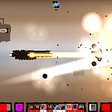# Build an isometric 3D game in 2D — #3 Make blocks move

This is part 3 of the 2d/3d(game/engine) conversion used in my puzzle game: Ladder Box, which is available on Steam:

You can find all the textures, scripts in this GitHub repo: https://github.com/fengjiongmax/3D_iso_in_2D

Godot 3.2.x is used in this project, you can check the commits for what’s new in each part of this series.

===

Before we make more changes to movable and unmovable scripts:

name movable Movable and add every movable to group “movable”:

`class_name Movableextends BlockBasefunc _ready(): add_to_group("movable") pass`

name unmovable Unmovable:

`class_name Unmovableextends BlockBasefunc _ready(): pass`

# Store the grid content

We need to know what’s on our board and their position, and a place that stores this information, I’m putting this in the global Grid(grid.gd).

To make things more organized, let’s move something that can be calculated statically into another script.

Create a script call grid_utils.gd:

`class_name GridUtilsconst TEXTURE_SCALE = 3const texture_w := 24const texture_h := 25const SINGLE_X = Vector2(texture_w/2,texture_h/4) * TEXTURE_SCALEconst SINGLE_Z = Vector2(-texture_w/2,texture_h/4) * TEXTURE_SCALEconst SINGLE_Y = Vector2(0,-texture_h/2) * TEXTURE_SCALEstatic func game_to_engine(x:int,y:int,z:int) -> Vector3: var _rtn_2d = Vector2.ZERO _rtn_2d += x*SINGLE_X _rtn_2d += z*SINGLE_Z _rtn_2d += y*SINGLE_Y var _z = (x+y+z)*2 return Vector3(_rtn_2d.x,_rtn_2d.y,_z)static func game_to_enginev(game_pos:Vector3) -> Vector3: return game_to_engine(int(game_pos.x),int(game_pos.y),int(game_pos.z))static func calc_xyz(v3:Vector3) -> int: return int(v3.x+v3.y+v3.z)static func game_direction_to_engine(direction:Vector3) -> Vector2: match direction:  Vector3.FORWARD:   return -SINGLE_Z  Vector3.BACK:   return SINGLE_Z  Vector3.LEFT:   return -SINGLE_X  Vector3.RIGHT:   return SINGLE_X  Vector3.UP:   return SINGLE_Y  Vector3.DOWN:   return -SINGLE_Y return Vector2.ZERO`

then grid.gd after clean up and adding some function related to the grid content( which can not be accessed by static function):

`# Godot Global/Autoload : Gridextends Nodevar game_arr = [] # a 3D array# let's just assume we'll have a board with the size of V3(8,8,8)var game_size = Vector3(8,8,8)func _ready(): set_grid_array()func set_grid_array(): game_arr.clear() for x in range(game_size.x):  game_arr.append([])  for y in range(game_size.y):   game_arr[x].append([])   for _z in range(game_size.z):    game_arr[x][y].append(null)func get_game_axis(x,y,z) -> Object: if !coordinate_within_range(x,y,z):  return Object()  return game_arr[x][y][z]func get_game_axisv(pos:Vector3) -> Object: return get_game_axis(pos.x,pos.y,pos.z)func coordinate_within_range(x:int, y:int, z:int) -> bool: if x <0 || y<0 || z<0 || \ x >= len(game_arr) || y >= len(game_arr) || z >= len(game_arr):  return false return truefunc coordinate_within_rangev(pos:Vector3) -> bool: return coordinate_within_range(int(pos.x),int(pos.y),int(pos.z))func set_axis_obj(obj:Object, x:int, y:int, z:int) -> void: if coordinate_within_range(x,y,z):  game_arr[x][y][z] = objfunc set_axis_objv(obj:Object,pos:Vector3) -> void: set_axis_obj(obj,int(pos.x),int(pos.y),int(pos.z))`
• ‘game_arr’ is the variable that stores our grid content.
• the board size is V3(8,8,8)

Change ‘set_game_pos’ in block_base.gd :

`class_name BlockBaseextends Spritevar game_pos:Vector3func _ready(): passfunc set_game_pos(x:int,y:int,z:int): game_pos = Vector3(x,y,z) var engine_pos = GridUtils.game_to_engine(x,y,z) self.position = Vector2(engine_pos.x,engine_pos.y) self.z_index = engine_pos.z passfunc set_game_posv(new_pos:Vector3): set_game_pos(int(new_pos.x),int(new_pos.y),int(new_pos.z)) pass`

# Finite State Machine

We will be using a finite state machine to make those blocks move the way we want. You can learn about the concept of FSM here.

First, we need to define the states for those movable blocks.

## 1. Idle

This is the initial state for our movables, and when they end their movement standing still.

## 2. Move

When we issue commands to make them move in four directions.

## 3. Jump

When blocks meet one block higher than their current game_pos.y, they will enter this state, and then they will continue moving in their direction, or fall to their previous position.

## 4. Fall

Fall to game-pos.y - 1.

======

That’s all the states for movable. In this part, we’ll get idle and move states work.

# Code for FSM

Let’s first create scripts for state machine and state base class.

state_base.gd:

`class_name StateBaseextends Nodeonready var _stae_machine := get_parent()var movable:Movable# then we can minipulate our movable in our statesfunc _ready(): movable = owner as Movable pass# we receive commands from our main scene# in our senario, we will not handle use input in our statesfunc _command(_msg:Dictionary={}) -> void: passfunc _update(_delta:float) -> void: pass# when entering the states,we run this functionfunc _enter(_msg:={}) -> void: pass# run this when states exitfunc _exit() -> void: pass`

state_machine.gd:

`class_name StateMachineextends Nodeexport var initial_state := NodePath()onready var state: StateBase = get_node(initial_state) setget set_stateonready var _state_name := state.namevar movable:Movablefunc _ready(): state._enter() movable = owner as Movable passfunc _update(delta) -> void : state._update(delta)func receive_command(msg:Dictionary) -> void: state._command(msg) passfunc switch_state(target_state_path:String,msg:Dictionary ={}) -> void: if ! has_node(target_state_path):  return  var target_state := get_node(target_state_path)  state._exit() self.state = target_state state._enter(msg)func set_state(value: StateBase) -> void: state = value _state_name = state.name pass`

create nodes for states in the movable scene:

In the inspector, assign the initial state to ‘idle’.

and attach scripts to all those states that extend our StateBase class:

`extends StateBase`

# Send command to movable

We’re gonna use ‘a,s,z,x’ to navigate our movable:

Let’s handle input in our main scene, then send command to movable to make them from idle to other states.

And add the function to handle input to send the command, in main.gd:

`func _input(event): if event.is_action_pressed("movable_forward"):  send_command(Vector3.FORWARD) elif event.is_action_pressed("movable_back"):  send_command(Vector3.BACK) elif event.is_action_pressed("movable_left"):  send_command(Vector3.LEFT) elif event.is_action_pressed("movable_right"):  send_command(Vector3.RIGHT)func send_command(command:Vector3) -> void: for _m in get_tree().get_nodes_in_group("movable"):  _m.receive_command({"direction":command}) set_physics_process(true)`

In idle.gd:

`extends StateBasefunc _command(_msg:Dictionary={}) -> void: if _msg.keys().has("direction"):  _state_machine.switch_state("move",{"direction":_msg["direction"]})  pass pass`

Now we switch to the move state once we send the command in idle state, if we add something in _enter function of move.gd:

`extends StateBasevar direction: Vector3func _enter(_msg:Dictionary={}) -> void: if !_msg.keys().has("direction"):  return  direction = _msg["direction"] print("enter move")`

And run the scene, you should see the output :)

At the _enter function of the move.gd, we will calculate how the movable move in the engine by the given direction, also the “next position” when the movable moved one block along that direction, I choose to make the calculation in a separate function called set_next_target, the “next” related variables will have a prefix “target”.

Now edit the move.gd:

`extends StateBasevar direction: Vector3var engine_direction:Vector2var target_game_pos:Vector3var target_z:intvar target_engine_pos:Vector2func _enter(_msg:Dictionary={}) -> void: if !_msg.keys().has("direction"):  return  direction = _msg["direction"] engine_direction = GridUtils.game_direction_to_engine(direction)set_next_target()func set_next_target(): target_game_pos = movable.game_pos + direction var _target_game_pos_obj = Grid.get_game_axisv(target_game_pos)  if Grid.coordinate_within_rangev(target_game_pos) && _target_game_pos_obj == null:  var _target_v3 = GridUtils.game_to_enginev(target_game_pos)  target_engine_pos = Vector2(_target_v3.x,_target_v3.y)  target_z = _target_v3.z  movable.set_game_posv(target_game_pos)  if direction == Vector3.FORWARD || direction == Vector3.LEFT:   movable.z_index = target_z + 1  else:   movable.z_index = target_z - 1 else:  _state_machine.switch_state("idle")  pass`

Run the scene and hit z:

it moved.

But we don’t want them to move to the next block at once, we want them to move to their target gradually.

Edit block_base.gd:

`class_name BlockBaseextends Spritevar game_pos:Vector3 = Vector3.INFfunc _ready():# print(game_pos) passfunc initial_game_pos(x:int,y:int,z:int) -> void: set_game_pos(x,y,z) engine_fit_game_pos()func initial_game_posv(new_game_pos:Vector3) -> void: initial_game_pos(int(new_game_pos.x),int(new_game_pos.y),int(new_game_pos.z))func engine_fit_game_pos(): var engine_pos = GridUtils.game_to_enginev(game_pos) self.position = Vector2(engine_pos.x,engine_pos.y) self.z_index = engine_pos.z passfunc set_game_pos(x:int,y:int,z:int) -> void: if game_pos != Vector3.INF:  Grid.set_axis_objv(null,game_pos) Grid.set_axis_obj(self, x, y, z) game_pos = Vector3(x,y,z)func set_game_posv(new_game_pos:Vector3) ->void: set_game_pos(int(new_game_pos.x),int(new_game_pos.y),int(new_game_pos.z))`
• separate the original set_game_pos into two functions

and change new_movable and new_unmovable in main.gd:

`func new_movable(x,y,z): var _m = movable.instance() \$movable.add_child(_m) _m.initial_game_pos(x,y,z) passfunc new_unmovable(x,y,z): var _u = unmovable.instance() \$unmovable.add_child(_u) _u.initial_game_pos(x,y,z) pass`

change set_game_pos to initial_game_pos.

Then we need to make movable moves in _update function in the move.gd, but before we do that, we know the blocks move in a Vector 2 direction, and they are moving in a grid, but the distance of each update may vary, so after an update, the result of the movement may be:

• haven’t reached target_engine_pos yet.
• movable.position = target_engine_pos
• movable has moved over the target_engine_pos.

So in the last two situations, both should be labeled movable has reached the target position, let’s create a script to help us do this:

In math.gd:

`class_name Math# start < target < endstatic func is_between(start:float,end:float,target:float) -> bool: var _start = round(start * 10)/10 var _end = round(end * 10)/10 var _target = round(target * 10)/10  var _tmp  if _start >= _end:  _tmp = _start  _start = _end  _end = _tmp  if _start <= _target && target <= _end:  if _end - _start >= _end - _target:   return true  return falsestatic func is_betweenv(start:Vector2,end:Vector2,target:Vector2) -> bool: return is_between(start.x,end.x,target.x) &&\  is_between(start.y,end.y,target.y)`

Before we edit move.gd, we can define a constant in movable.gd to define the move speed, I’m setting this to 2( this is slow but you can see the movement):

`const MOVESPEED = 2`

Then we can add the _update function in the move.gd:

`func _update(_delta:float) -> void: var _after_move = movable.position + engine_direction * _delta * movable.MOVESPEED var _reach_target = Math.is_betweenv(movable.position,_after_move,target_engine_pos)  if !_reach_target:  movable.position = _after_move else:  movable.position = target_engine_pos  movable.z_index = target_z`

Run the scene:

The gif is slower than the running scene, but it works as expected.

That's it for this part, you can check out the Github repo for the code committed, to make it work as in Ladder Box, we still have a long way to go.

Stay tuned!

--

--

--

## More from Fj max

im404.me

Love podcasts or audiobooks? Learn on the go with our new app.

## Experience with LSMSOC…## Rabin-Karp Algorithm Using Polynomial Hashing and Modular Arithmetic## An Interview with Antonio Leiva, Author of Kotlin for Android Developers## Learning the basic of OOP with Python and Testing with Pythons built-in Unittest## How to Load a CSV to a Dataframe with Newline Characters Using Apache Spark and Databricks## Alvin’s Dew Drop Daily — Issue #104im404.me

## Building a Game in a Month## Indie Game Development: What Genre Should I Make? -## Aivaras Klimas is chasing his game developer dream in Lithuania | Stories About Indie Devs## Centralization vs Decentralization